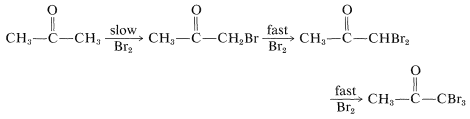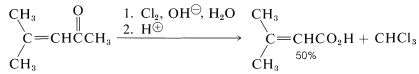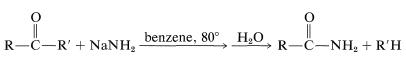# 17.3: Halogenation of Aldehydes and Ketones

$$\newcommand{\vecs}{\overset { \rightharpoonup} {\mathbf{#1}} }$$ $$\newcommand{\vecd}{\overset{-\!-\!\rightharpoonup}{\vphantom{a}\smash {#1}}}$$$$\newcommand{\id}{\mathrm{id}}$$ $$\newcommand{\Span}{\mathrm{span}}$$ $$\newcommand{\kernel}{\mathrm{null}\,}$$ $$\newcommand{\range}{\mathrm{range}\,}$$ $$\newcommand{\RealPart}{\mathrm{Re}}$$ $$\newcommand{\ImaginaryPart}{\mathrm{Im}}$$ $$\newcommand{\Argument}{\mathrm{Arg}}$$ $$\newcommand{\norm}{\| #1 \|}$$ $$\newcommand{\inner}{\langle #1, #2 \rangle}$$ $$\newcommand{\Span}{\mathrm{span}}$$ $$\newcommand{\id}{\mathrm{id}}$$ $$\newcommand{\Span}{\mathrm{span}}$$ $$\newcommand{\kernel}{\mathrm{null}\,}$$ $$\newcommand{\range}{\mathrm{range}\,}$$ $$\newcommand{\RealPart}{\mathrm{Re}}$$ $$\newcommand{\ImaginaryPart}{\mathrm{Im}}$$ $$\newcommand{\Argument}{\mathrm{Arg}}$$ $$\newcommand{\norm}{\| #1 \|}$$ $$\newcommand{\inner}{\langle #1, #2 \rangle}$$ $$\newcommand{\Span}{\mathrm{span}}$$$$\newcommand{\AA}{\unicode[.8,0]{x212B}}$$

## Synthesis of $$\alpha$$-Halo Ketones

Halogenation of saturated aldehydes and ketones usually occurs exclusively by replacement of hydrogens alpha to the carbonyl group:The reagents that commonly are used to halogenate carbonyl compounds are those that are used to halogenate alkanes (e.g. $$\ce{Cl_2}$$, $$\ce{Br_2}$$, $$\ce{SO_2Cl_2}$$, and N-bromoamides; see Sections 4-4 and 14-3). However, the characteristics of the two types of halogenation normally are very different. 2-Propanone has been particularly well studied, and the important features of the halogenation of this compound are summarized as follows:

1. 2-Propanone reacts easily with chlorine, bromine, and iodine.
2. 2-Propanone reacts at the same rate with each halogen. Indeed, the rate of formation of the 1-halo-2-propanone is independent of the concentration of the halogen, even at very low halogen concentrations.
3. The halogenation of 2-propanone is catalyzed by both acids and bases. The rate expressions for formation of 1-halo-2-propanone in water solution are:
• at moderate $$\ce{OH}^\ominus$$ concentrations

$v = k \left[ \ce{CH_3COCH_3} \right] \left[ \ce{OH}^\ominus \right]$

• at moderate $$\ce{H}^\oplus$$ concentrations

$v = k' \left[ \ce{CH_3COCH_3} \right] \left[ \ce{H}^\oplus \right]$

The ratio of $$k$$ to $$k'$$ is 12,000, which means that hydroxide ion is a much more effective catalyst than is hydrogen ion.

The hydroxide ion is a much more effective catalyst than is hydrogen ion

To account for the role of the catalysts and the independence of the rate from the halogen concentration, the ketone necessarily must be slowly converted by the catalysts to something that can react rapidly with halogen to give the products. This something is either the enol or the enolate anion of 2-propanone:As long as the first step is slow compared with the steps of Equations 17-2 and 17-3, the overall rate of reaction will be independent of both the concentration of halogen and whether it is chlorine, bromine, or iodine (cf. Section 4-4C).

The reaction of either the enol or the enolate anion (Equations 17-2 or 17-3) with $$\ce{Br_2}$$ resembles the first step in the electrophilic addition of halogens to carbon-carbon multiple bonds (Section 10-3A). However, the second step, addition of the nucleophilic halide, if it occurs at all, does not produce any stable product:Unsymmetrical ketones, such as 2-butanone, can form two different enols that will react with halogens to give isomeric halo ketones:The composition of the product mixture will depend on the relative rates of formation of the isomeric enols, provided that the halogenation step is not a reversible reaction. Barring any serious steric effects that influence the rate of reaction, the more rapidly formed enol generally is the more thermodynamically stable enol.

## The Haloform Reaction

The previous discussion of the halogenation of ketones is incomplete in one important respect concerning base-induced halogenation. That is, once an $$\alpha$$-halo ketone is formed, the other hydrogens on the same carbon are rendered more acidic by the electron-attracting effect of the halogen and are replaced much more rapidly than the first hydrogen:The result is that, if the monobromoketone is desired, the reaction is carried out best with an acidic catalyst rather than a basic catalyst. A further complication in the base-catalyzed halogenation of a methyl ketone is that the trihaloketone formed is attacked readily by base, thereby resulting in cleavage of a carbon-carbon bond:This sequence is called the haloform reaction because it results in the production of chloroform, bromoform, or iodoform, depending upon the halogen used. The haloform reaction is a useful method for identification of methyl ketones, particularly when iodine is used, because iodoform is a highly insoluble, bright-yellow solid. The reaction also is very effective for the synthesis of carboxylic acids when the methyl ketone is more available than the corresponding acid:Because the haloform reaction is fast, in some cases it can be used to prepare unsaturated acids from unsaturated ketones without serious complications caused by addition of halogen to the double bond:A reaction somewhat similar to the cleavage of haloforms with hydroxide occurs with ketones that do not have $$\alpha$$-hydrogens through the action of sodium amide:This reaction, called the Haller-Bauer reaction, has utility for the preparation of amides of the types $$\ce{ArCONH_2}$$ and tert-$$\ce{RCONH_2}$$, and, through hydrolysis, the corresponding carboxylic acids.

## Reactions of $$\alpha$$-Halo Ketones

The halogen of an $$\alpha$$-halo aldehyde or an $$\alpha$$-halo ketone is exceptionally unreactive in $$S_\text{N}1$$-displacement reactions, but is exceptionally reactive in $$S_\text{N}2$$ displacements, compared with the halogen of alkyl halides having comparable potential steric effects. Similar behavior is observed with $$\alpha$$-halo carboxylic acids and is discussed in Chapter 18.In some circumstances, the production of a 2-halo alcohol by reduction of the carbonyl group of an $$\alpha$$-halo ketone with metal hydrides is a useful synthetic reaction:When one attempts $$E2$$ reactions with $$\alpha$$-halo ketones using strong bases such as alkoxides, an interesting rearrangement pathway may occur called the Favorskii rearrangement. In this reaction, the $$\alpha$$-halo ketone is converted to an ester. For example, 2-chlorocyclohexanone is converted to the methyl ester of cyclopentanecarboxylic acid by treatment with sodium methoxide in ether:The mechanism of this reaction has been the subject of many investigations.